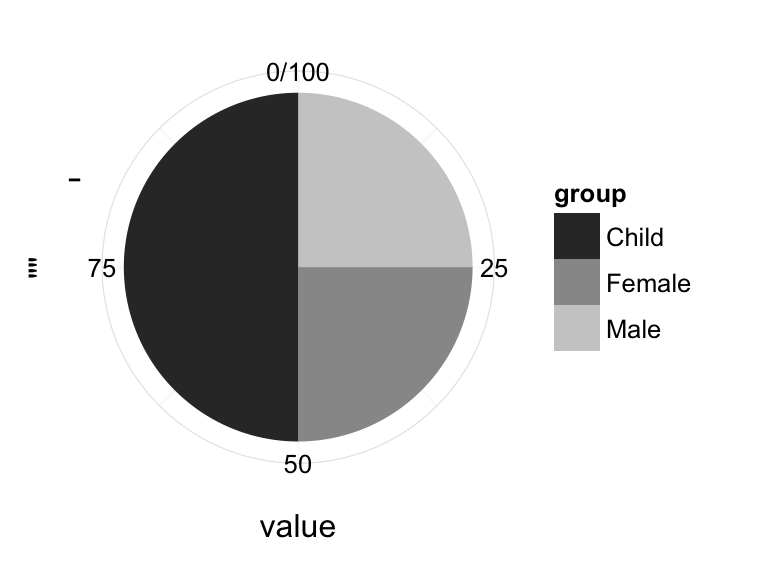# ggplot2 pie chart : Quick start guide - R software and data visualization

This R tutorial describes how to create a pie chart for data visualization using R software and ggplot2 package.

The function coord_polar() is used to produce a pie chart, which is just a stacked bar chart in polar coordinates.

# Simple pie charts

Create some data :

``````df <- data.frame(
group = c("Male", "Female", "Child"),
value = c(25, 25, 50)
)
``````##    group value
## 1   Male    25
## 2 Female    25
## 3  Child    50``````

Use a barplot to visualize the data :

``````library(ggplot2)
# Barplot
bp<- ggplot(df, aes(x="", y=value, fill=group))+
geom_bar(width = 1, stat = "identity")
bp``````Create a pie chart :

``````pie <- bp + coord_polar("y", start=0)
pie``````# Change the pie chart fill colors

It is possible to change manually the pie chart fill colors using the functions :

• scale_fill_manual() : to use custom colors
• scale_fill_brewer() : to use color palettes from RColorBrewer package
• scale_fill_grey() : to use grey color palettes
``````# Use custom color palettes
pie + scale_fill_manual(values=c("#999999", "#E69F00", "#56B4E9"))````````````# use brewer color palettes
pie + scale_fill_brewer(palette="Dark2")````````````pie + scale_fill_brewer(palette="Blues")+
theme_minimal()````````````# Use grey scale
pie + scale_fill_grey() + theme_minimal()``````Read more on ggplot2 colors here : ggplot2 colors

# Create a pie chart from a factor variable

PlantGrowth data is used :

``head(PlantGrowth)``
``````##   weight group
## 1   4.17  ctrl
## 2   5.58  ctrl
## 3   5.18  ctrl
## 4   6.11  ctrl
## 5   4.50  ctrl
## 6   4.61  ctrl``````

Create the pie chart of the count of observations in each group :

``````ggplot(PlantGrowth, aes(x=factor(1), fill=group))+
geom_bar(width = 1)+
coord_polar("y")``````# Customized pie charts

Create a blank theme :

``````blank_theme <- theme_minimal()+
theme(
axis.title.x = element_blank(),
axis.title.y = element_blank(),
panel.border = element_blank(),
panel.grid=element_blank(),
axis.ticks = element_blank(),
plot.title=element_text(size=14, face="bold")
)``````
1. Apply the blank theme
2. Remove axis tick mark labels
3. Add text annotations : The package scales is used to format the labels in percent
``````# Apply blank theme
library(scales)
pie + scale_fill_grey() +  blank_theme +
theme(axis.text.x=element_blank()) +
geom_text(aes(y = value/3 + c(0, cumsum(value)[-length(value)]),
label = percent(value/100)), size=5)````````````# Use brewer palette
pie + scale_fill_brewer("Blues") + blank_theme +
theme(axis.text.x=element_blank())+
geom_text(aes(y = value/3 + c(0, cumsum(value)[-length(value)]),
label = percent(value/100)), size=5)``````# Infos

This analysis has been performed using R software (ver. 3.1.2) and ggplot2 (ver. 1.0.0)